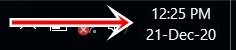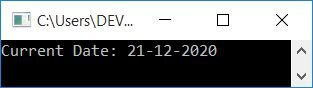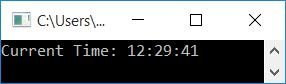# C++ program to print date and time

In this article, you will learn and get code to print date and time in default and custom formats using a C++ program. Here is the list of programs available in this article:

## Print the current date and time in the standard format

This program prints the current date and time in the default format. The question is, "Write a program in C++ to print date and time." Here is its answer:

```#include<iostream>
#include<ctime>
using namespace std;
int main()
{
time_t tmNow = time(0);
char *dt = ctime(&tmNow);
cout<<"Current Date/Time: "<<dt;
cout<<endl;
return 0;
}```

This program was built and runs under the Code::Blocks IDE. Here is its sample output:As you can see, this program produces the current local date and time in its default format. Here is the snapshot, which shows the local date and time in my computer system right now (at the same time while executing the above program):In this program, the time_t is not a primitive (primary) data type. It is defined to store a system's time value. The system's time values are returned from time(), a standard library function. So the following C++ statement:

`time_t tmNow = time(0);`

initializes the number of seconds elapsed since 00:00 hours, Jan 1, 1970 UTC. That is, after executing the above statement, the variable, tmNow holds the total number of seconds elapsed since 1st January, 1970. The number of seconds since then is approximately 1608536102.

If you want to convert this number of seconds into years, then here is a step-by-step conversion:

```1608536102 seconds = (1608536102/60) minutes
= 26808935 minutes
= (26808935/60) hours
= 446815 hours
= (446815/24) days
= 18617 days
= (18617/365.24) years
= 50.97 years```

So if we add 50.97 years to 1970, we'll get 1970+50.97, or 2020.97. That is almost equal to 2021. The date when this program is executed.

Note: To satisfy this condition, we've added 1970 to the year in every program given below.

Note: To understand why I've divided by 365.24 instead of 365 to convert days into years, refer to  Leap Year Formula Explained to get every required detail about the topic.

The function ctime() returns a string that represents the local time. This function is defined in the ctime header file.

## Print the current date in DD-MM-YYYY format

Because the previous program prints dates in default format, let's print the dates first in our required format. Later on, I wrote a program to print time in a custom format.

```#include<iostream>
#include<ctime>
using namespace std;
int main()
{
time_t tmNow;
tmNow = time(NULL);
struct tm t = *localtime(&tmNow);
cout<<"Current Date: "<<t.tm_mday<<"-"<<t.tm_mon+1<<"-"<<t.tm_year+1900;
cout<<endl;
return 0;
}```

Here is its sample output:In the above program, the function localtime() returns the local time of the system. It is defined in the ctime header file. It accepts a parameter that represents the pointer to the time_t object and returns a pointer to a struct tm object. Now using the structure, the object tm can access the following data members:

• tm_mday
• tm_mon
• tm_year

Note: Since by default, 0 indicates January, I've added 1 to tm_mon before printing the month number.

## Print the current time in the format HH:MM:SS

Now let's print the time in HH:MM:SS format. Here, HH indicates hours, MM indicates minutes, and SS indicates seconds.

```#include<iostream>
#include<ctime>
using namespace std;
int main()
{
time_t tmNow;
tmNow = time(NULL);
struct tm t = *localtime(&tmNow);
cout<<"Current Time: "<<t.tm_hour<<":"<<t.tm_min<<":"<<t.tm_sec;
cout<<endl;
return 0;
}```

The snapshot given below shows the sample output produced by this program:## Print the current date and time in your preferred format

This is the last program that formats the date in custom form. That is, we've used switch() to match and print the month name instead of the month number.

```#include<iostream>
#include<ctime>
using namespace std;
int main()
{
time_t tmNow;
tmNow = time(NULL);
struct tm t = *localtime(&tmNow);
int mon;
cout<<"Today's Date: "<<t.tm_mday<<" ";
mon = t.tm_mon+1;
switch(mon)
{
case 1:
cout<<"Jan, ";
break;
case 2:
cout<<"Feb, ";
break;
case 3:
cout<<"Mar, ";
break;
case 4:
cout<<"Apr, ";
break;
case 5:
cout<<"May, ";
break;
case 6:
cout<<"June, ";
break;
case 7:
cout<<"July, ";
break;
case 8:
cout<<"Aug, ";
break;
case 9:
cout<<"Sept, ";
break;
case 10:
cout<<"Oct, ";
break;
case 11:
cout<<"Nov, ";
break;
case 12:
cout<<"Dec, ";
break;
}
cout<<t.tm_year+1900;
cout<<"\nToday's Time: ";
if(t.tm_hour>=12)
{
if(t.tm_hour==12)
cout<<"12";
else
cout<<t.tm_hour-12;
cout<<":"<<t.tm_min<<":"<<t.tm_sec<<" PM";
}
else
cout<<t.tm_hour<<":"<<t.tm_min<<":"<<t.tm_sec<<" AM";
cout<<endl;
return 0;
}```

Here is its sample output:#### The same program in different languages

C++ Quiz

« Previous Program Next Program »